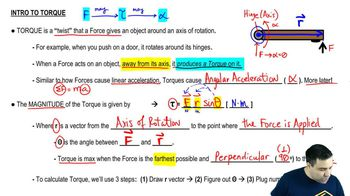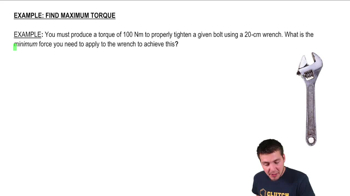Start typing, then use the up and down arrows to select an option from the list.
2:07 minutes
Problem 10b
Textbook Question

# A metal bar is in the xy-plane with one end of the bar at the origin. A force F = 97.00 N)i + (-3.00 N)j is applied to the bar at the point x = 3.00 m, y = 4.00 m. (a) In terms of unit vectors i and j, what is the position vector r for the point where the force is applied?Verified Solution
This video solution was recommended by our tutors as helpful for the problem above.
108views